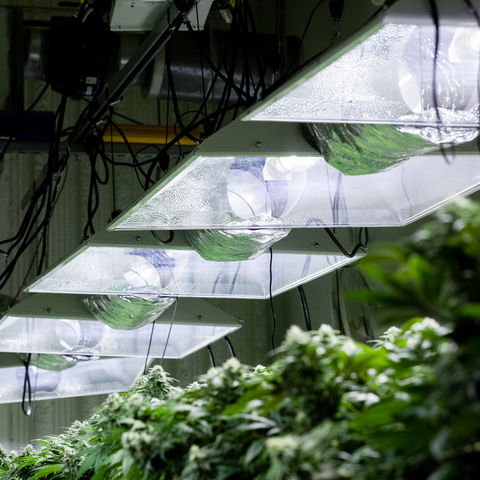# How Much Does It Cost To Run a Grow Light

Posted by Jeena Lugo on

Indoor growing has become increasingly popular over the past few years, with more and more people opting to grow their own plants, fruits, and vegetables indoors. One of the key components of indoor growing is the use of grow lights. Grow lights are designed to provide the necessary light spectrum for plants to grow and thrive indoors, especially in grow rooms or grow tents where natural light is limited. In this blog post, we will explore the cost of using grow lights for indoor growing and how to calculate the monthly cost.First, let's discuss the types of grow lights available in the market. There are several types of grow lights available, including high-intensity discharge (HID), fluorescent, and light-emitting diode (LED) grow lights. HID grow lights are the most popular and widely used grow lights for indoor growing. They are available in two types: metal halide (MH) and high-pressure sodium (HPS). Fluorescent grow lights are the second most popular grow lights, while LED grow lights are the newest addition to the market.

The cost of running a grow light depends on several factors, including the wattage of the light, the number of hours it is used per day, the electricity rate, and the energy cost per kilowatt-hour (kWh). The wattage of a grow light is the amount of power it uses, and it is usually measured in watts (W). For example, a 1,000-watt grow light uses 1,000 watts of power.

To calculate the cost to run a grow light, you need to know the electricity rate and the energy cost per kilowatt-hour. The electricity rate is the price you pay for electricity per unit, and it varies depending on your location and the electrical company you use. The energy cost per kilowatt-hour is the cost of using 1,000 watts of power for one hour, and it is usually expressed in cents.

Let's assume that you have a 1,000-watt grow light and you use it for 12 hours per day. The first step is to convert the wattage to kilowatts (kW) by dividing the wattage by 1,000. In this case, the grow light uses 1 kW of power per hour. If the energy cost per kilowatt-hour is 10 cents, the cost to run the grow light for one hour is 10 cents. Therefore, the cost to run the grow light for 12 hours per day is 1 kW x 12 hours x 10 cents = \$1.20 per day.

Now, let's calculate the monthly cost of using the grow light. To do this, you need to multiply the daily cost by the number of days in a month. Assuming there are 30 days in a month, the monthly cost of using the grow light for 12 hours per day is 30 x \$1.20 = \$36 per month.

It is worth noting that the cost of using a grow light can vary depending on the type of grow light you use. LED grow lights are more energy-efficient than HID and fluorescent grow lights, which means they use less power and cost less to run. LED grow lights also have a longer lifespan than other grow lights, which means they need to be replaced less frequently.

Another factor that can affect the cost of using a grow light is the size of your grow room or grow tent. A larger grow room or tent will require more grow lights, which means the cost of running them will be higher.

In addition to the cost of running the grow lights, there is also the initial cost of purchasing the grow light system. Grow light costs can vary widely depending on the type and size of the system you choose. LED grow lights tend to be more expensive than HID and fluorescent grow lights, but they also offer long-term savings due to their energy efficiency and longer lifespan.

When deciding on a grow light system, it is important to consider the specific needs of your plants and the size of your grow room or tent. Different plants have different light requirements, so it is important to choose a grow light that provides the appropriate light spectrum for your plants. It is also important to choose a grow light that provides enough coverage for your entire grow room or tent.

Overall, the cost of using a grow light for indoor growing can vary depending on several factors. These factors include the type of grow light, the wattage of the light, the number of hours it is used per day, the electricity rate, the energy cost per kilowatt-hour, and the size of your grow room or tent. By calculating these factors, you can estimate the monthly cost of using a grow light for indoor growing.

In conclusion, using a grow light for indoor growing can be an effective way to provide the necessary light spectrum for plants to grow and thrive. While there is a cost associated with using a grow light, it can be managed by choosing an energy-efficient grow light system and monitoring the number of hours the light is used per day. By taking these factors into consideration, you can successfully grow plants indoors without breaking the bank.